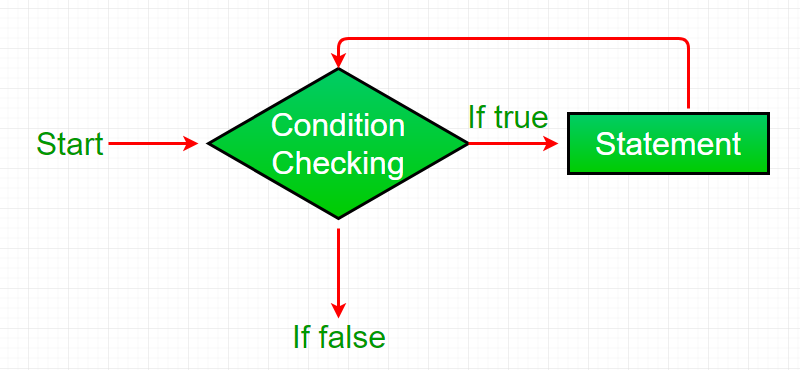Related Articles

# while loop in Perl

• Last Updated : 21 Nov, 2019

A while loop in Perl generally takes an expression in parenthesis. If the expression is True then the code within the body of while loop is executed. A while loop is used when we don’t know the number of times we want the loop to be executed however we know the termination condition of the loop. It is also known as an entry controlled loop as the condition is checked before executing the loop. The while loop can be thought of as a repeating if statement.

Syntax :

```while (condition)
{
# Code to be executed
}
```

Flow Chart:Example :

 `# Perl program to illustrate``# the while loop`` ` `# while loop``\$count` `= 3;``while` `(``\$count` `>= 0)``{``    ``\$count` `= ``\$count` `- 1;``    ``print` `"GeeksForGeeks\n"``;``}`

Output:

```GeeksForGeeks
GeeksForGeeks
GeeksForGeeks
GeeksForGeeks
```

### Nested while loop

A Nested while loop is one in which one while loop is used inside another while loop. In a Nested while loop, for each iteration of the outer while loop, the inner while loop gets executed completely.

 `#!/usr/bin/perl``# Perl program for Nested while loop``\$a` `= 0;  `` ` `# while loop execution  ``while``( ``\$a` `<= 2 )``{  ``    ``\$b` `= 0;  ``    ``while``( ``\$b` `<= 2 )``    ``{  ``        ``printf` `"\$a \$b\n"``;  ``        ``\$b``++;  ``    ``}  ``    ``\$a``++;  ``}  `

Output:

```0 0
0 1
0 2
1 0
1 1
1 2
2 0
2 1
2 2
```

### Infinite While Loop

While loop can execute infinite times which means there is no terminating condition for this loop. In other words, we can say there are some conditions that always remain true, which causes while loop to execute infinite times or we can say it never terminates.

Below program will print the specified statement infinite time and also gives the runtime error as Output Limit Exceeded on online IDE

 `# Perl program to illustrate``# the infinite while loop`` ` `# infinite while loop``# containing condition 1 ``# which is always true``while``(1)``{``    ``print` `"Infinite While Loop\n"``;``}`

Output:

```Infinite While Loop
Infinite While Loop
Infinite While Loop
Infinite While Loop
.
.
.
.
```

My Personal Notes arrow_drop_up